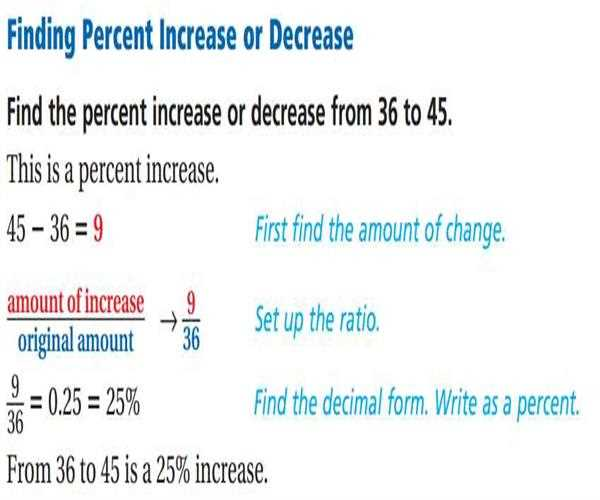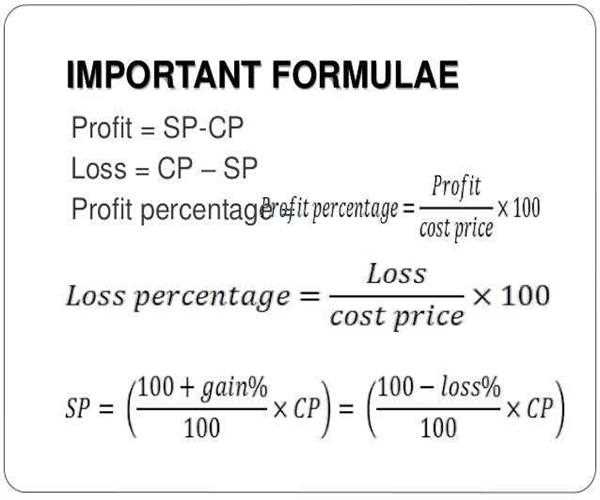# how to calculate percentage \$(function () { var favoriteStarId = "Q" + "51881"; var ExistsUrl = \$("#" + favoriteStarId).attr("ExistsUrl"); var favoriteData = { EntityId: "51881", EntityType: "Q", UserID: "0" } \$.ajax({ type: "POST", url: ExistsUrl, data: JSON.stringify(favoriteData), dataType: "json", contentType: 'application/json; charset=utf-8', success: function (response) { if (response.success == true) { if (response.isExist == true) { \$("#" + favoriteStarId).removeClass('qa-favorite-button'); \$("#" + favoriteStarId).addClass('qa-unfavorite-button'); } else { \$("#" + favoriteStarId).removeClass('qa-unfavorite-button'); \$("#" + favoriteStarId).addClass('qa-favorite-button'); } } } }); \$(".btn-fav").hover(function () { var classes = []; \$('.btn-fav').each(function () { classes.push(\$(this).attr('class')); }); if (classes == "btn-fav qa-favorite-button") { \$('.btn-fav').css("background-color", "#ffab0f"); } else { \$('.btn-fav').css("background-color", "#2f364a"); } \$('.btn-fav').css("background-position", "center bottom"); }, function () { var classes = []; \$('.btn-fav').each(function () { classes.push(\$(this).attr('class')); }); if (classes == "btn-fav qa-favorite-button") { \$('.btn-fav').css("background-color", "#2f364a"); } else { \$('.btn-fav').css("background-color", "#ffaf04"); } \$('.btn-fav').css("background-position", "center bottom"); }); })

 Remember: How to convert a percentage to a decimal Remove the percentage sign and divide by 100 15.6% = 15.6/100 = 0.156 Remember: How to convert a decimal to a percentage Multiply by 100 and add a percentage sign 0.876 = 0.876 * 100 = 87.6% Y of what is P percent? Written as an equation: Y / X = P% The 'what' is X that we want to solve for Multiply both sides by X to get X out of the denominator (Y / X) * X = P% * X becomes Y = P% * X Divide both sides by P% so that X is on one side of the equation Y ÷ P% = (P% * X) ÷ P% becomes Y ÷ P% = X Solution: Solve for X using the percentage formula X = Y ÷ P% Example: 4 of what is 12%? Written using the formula: X = 4 ÷ 12% Solve for X: X = Y ÷ P% Convert the percent to a decimal 12% ÷ 100 = 0.12 X = 4 ÷ 0.12 X = 33.3333 4 of 33.3333 is 12% What of X is P percent? Written as an equation: Y / X = P% The 'what' is Y that we want to solve for Multiply both sides by X to get Y on one side of the equation (Y ÷ X) * X = P% * X becomes Y = P% * X Solution: Solve for Y using the percentage formula Y = P% * X Example: What of 25 is 11%? Written using the formula: Y = 11% * 25 Convert the percent to a decimal 11% ÷ 100 = 0.11 Y = 0.11 * 25 Y = 2.75 So 2.75 of 25 is 11% Y of X is what percent? Written as an equation: Y / X = P% The 'what' is P% that we want to solve for Solution: Solve for P% using the percentage formula P% = Y / X Example: 9 of 13 is what percent? Written using the formula: P% = Y / X 9 ÷ 13 = P% 9 ÷ 13 = 0.6923 Convert decimal to percent by multiplying by 100 0.6923 * 100 = 69.23% 9 ÷ 13 = 69.23% So 9 of 13 is 69.23% What is P percent of X? Written as an equation: Y = P% * X The 'what' is Y that we want to solve for Remember to first convert percentage to decimal, dividing by 100 Solution: Solve for Y using the percentage formula Y = P% * X Example: What is 10% of 25? Written using the percentage formula: Y = 10% * 25 First convert percentage to a decimal 10/100 = 0.1 Y = 0.1 * 25 = 2.5 So 10% of 25 is 2.5